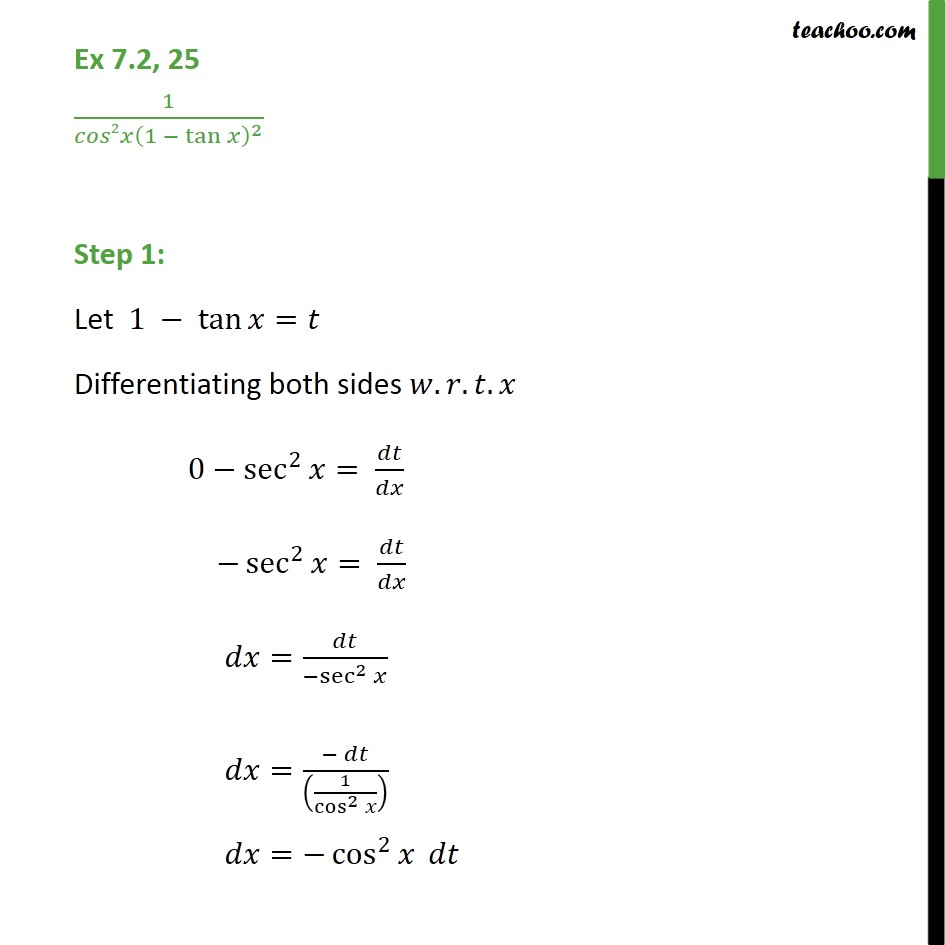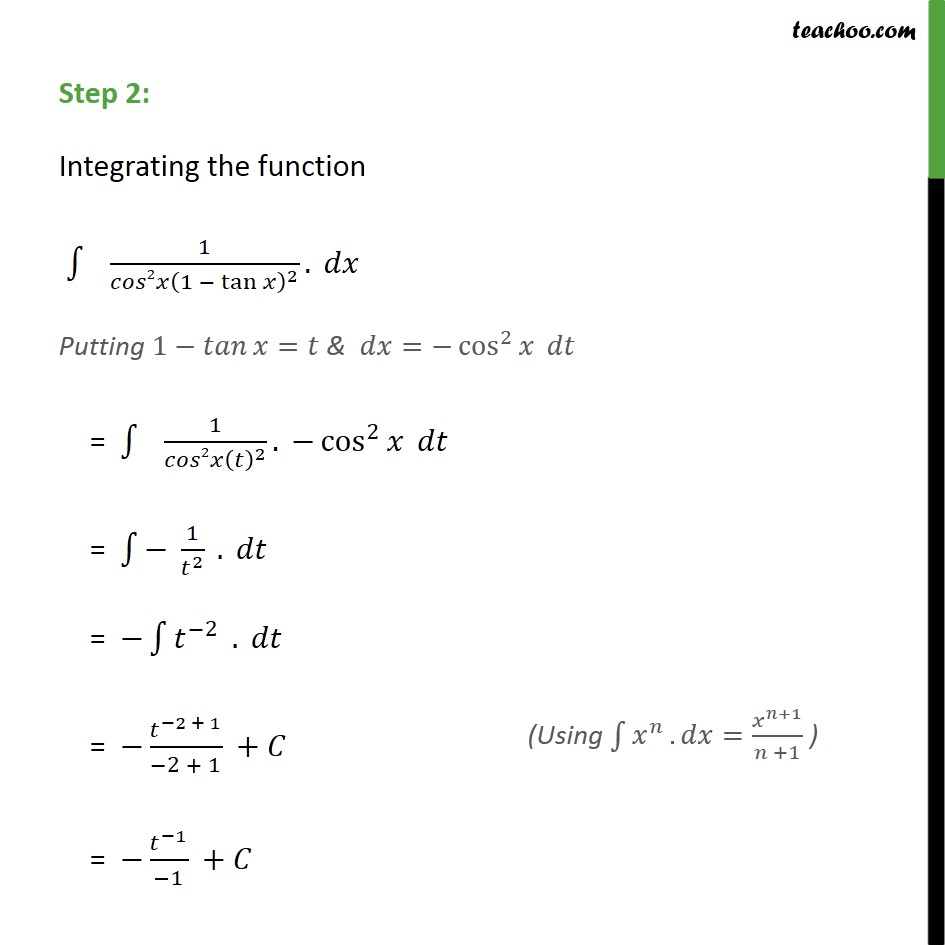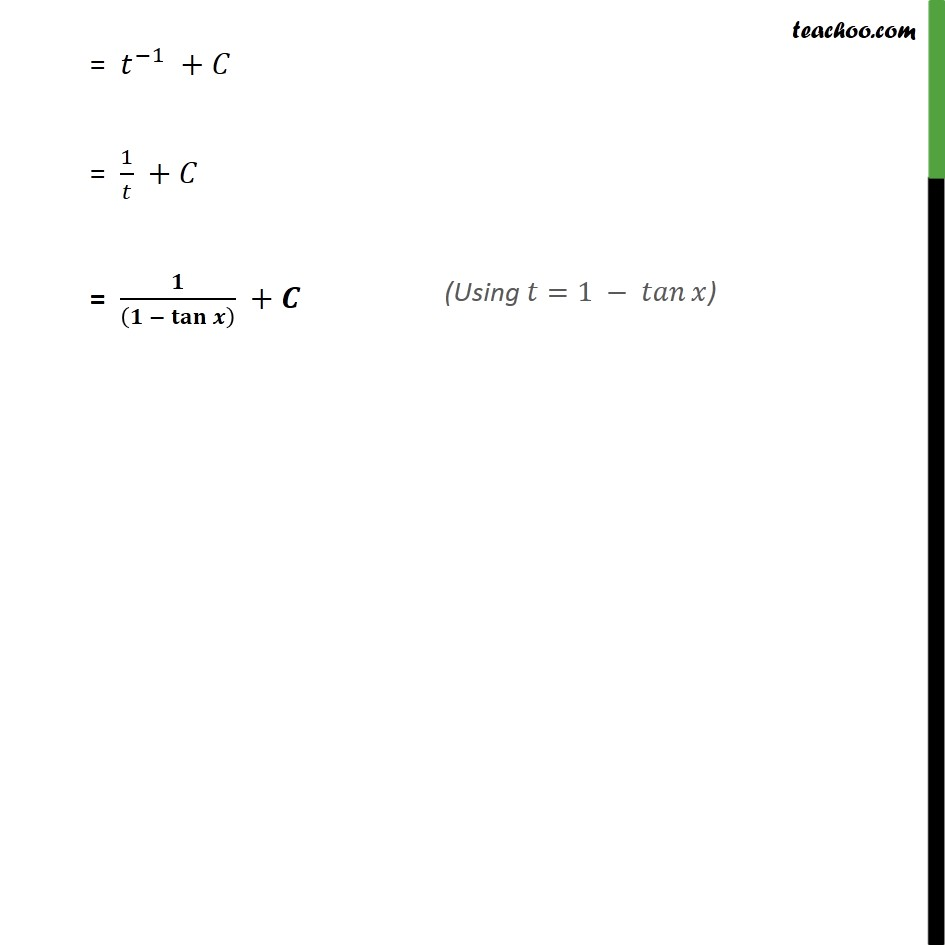1. Chapter 7 Class 12 Integrals (Term 2)
2. Serial order wise
3. Ex 7.2

Transcript

Ex 7.2, 25 1 2 1 tan 2 Step 1: Let 1 tan = Differentiating both sides . . . 0 sec 2 = sec 2 = = sec 2 = 1 cos 2 = cos 2 Step 2: Integrating the function 1 2 1 tan 2 . Putting 1 = & = cos 2 = 1 2 2 . cos 2 = 1 2 . = 2 . = 2 + 1 2 + 1 + = 1 1 + = 1 + = 1 + = +

Ex 7.2